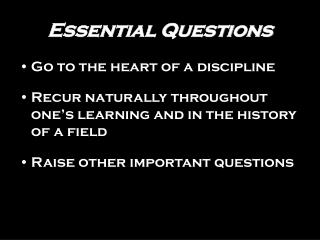# Essential Questions - PowerPoint PPT PresentationDownload PresentationEssential Questions

Essential Questions
Download Presentation## Essential Questions

- - - - - - - - - - - - - - - - - - - - - - - - - - - E N D - - - - - - - - - - - - - - - - - - - - - - - - - - -
##### Presentation Transcript

1. Essential Questions • Go to the heart of a discipline • Recur naturally throughout one’s learning and in the history of a field • Raise other important questions

2. Essential Questions Examples • Must a story have a moral, heroes, and villains? • How does an organism’s structure enable it to survive in its environment? • Who is a friend? • Do we always mean what we say and say what we mean?

3. More Essential Questions • What makes a video game “good”? • Where do Waves Come From? • When is Fighting Good and When Is it Bad? • What is a Home? • Who Owns the Internet?

4. Unit Questions • Provide subject- and topic- specific doorways to essential questions • Have no obvious “right” answer • Are deliberately framed to provoke and sustain student interest

5. Unit Questions Examples • Is Huck Finn a hero? • Has history proven that the enemy of my enemy is my friend? • How do cats see in the dark? • Does technology always lead to progress? • Is the gap between rich and poor better now than 100 years ago?

6. Essential Question Are vacations worth the cost?

7. Unit Questions • What is the greatest expense of traveling abroad? • Which country would be the most interesting to visit? • What is the best travel method for seeing a country? • How would you estimate the cost of traveling in Germany?

8. Unit Question Are Germany’s gas prices affordable?

9. Joe’s Trip to Germany By Richard Holden

10. Statement of Problem • Joe is planning a trip to Germany • He will rent a car to drive while there • He knows the places he plans to go • He wants to know how much he will spend on gas while there (in U.S. dollars)

11. Joe Quantifies the Information • Know the total distance to drive • Know the price of gasoline in Germany • Know how many miles/gallon he gets • Know the conversions: • For kilometers to miles • For gallons to liters • For Deutsch Marks to dollars

12. Total Distance to Drive Joe studies a map of Germany and finds he will drive a total of 6500 kilometers.

13. Price of Gas in Germany • Joe finds out from an Internet search that the price of gasoline in Germany is 1.6 DM per liter (they are still accepting Deutsche Marks in Germany)

14. Miles Per Gallon Joe’s car gets 32 miles per gallon

15. Conversions • 1 Km = 0.62 miles • 1 gal = 3.785 liters • 1.99 DM = \$1.00

16. Distance is 6500 Km Gas price is 1.6 DM/liter Car gets 22 mpg 1 Km = 0.62 miles 1 gal = 3.785 liters 1.99 DM = \$1.00 What is Now Known

17. The Secret!!! • Use ratios equal to one (identity property of multiplication) • Set up each ratio so units will cross cancel • Begin with the distance (6500 km) • Continue until the distance is changed to dollars

18. Distance is 6500 Km Gas price is 1.6 DM/liter Car gets 22 mpg 1 Km = 0.62 miles 1 gal = 3.785 liters 1.99 DM = \$1.00 What is Now Known  The Solution 6500 Km Next: Find an equation with Km in it

19. Distance is 6500 Km Gas price is 1.6 DM/liter Car gets 22 mpg 1 Km = 0.62 miles 1 gal = 3.785 liters 1.99 DM = \$1.00 What is Now Known  The Solution 6500 Km X 0.62 mi 1 Km Next: Find an equation with miles in it

20. Distance is 6500 Km Gas price is 1.6 DM/liter Car gets 22 mpg 1 Km = 0.62 miles 1 gal = 3.785 liters 1.99 DM = \$1.00 What is Now Known  The Solution 6500 Km X 0.62 mi X 1 gal 1 Km 22 mi Next: Find an equation with gallons in it

21. Distance is 6500 Km Gas price is 1.6 DM/liter Car gets 22 mpg 1 Km = 0.62 miles 1 gal = 3.785 liters 1.99 DM = \$1.00 What is Now Known  The Solution 6500 Km X 0.62 mi X 1 gal X 3.785 liters 1 Km 22 mi 1 gal Next: Find an equation with liters in it

22. Distance is 6500 Km Gas price is 1.6 DM/liter Car gets 22 mpg 1 Km = 0.62 miles 1 gal = 3.785 liters 1.99 DM = \$1.00 What is Now Known  The Solution 6500 Km X 0.62 mi X 1 gal X 3.785 liters X 1.6 DM 1 Km 22 mi 1 gal 1 liter Next: Find an equation with DM in it

23. Distance is 6500 Km Gas price is 1.6 DM/liter Car gets 22 mpg 1 Km = 0.62 miles 1 gal = 3.785 liters 1.99 DM = \$1.00 What is Now Known The Solution 6500 Km X 0.62 mi X 1 gal X 3.785 liters X 1.6 DM X \$1.00 1 Km 22 mi 1 gal 1 liter 1.99DM Finally, multiply by the numerators and then divide by the denominators.

24. Distance is 6500 Km Gas price is 1.6 DM/liter Car gets 22 mpg 1 Km = 0.62 miles 1 gal = 3.785 liters 1.99 DM = \$1.00 What is Now Known The Solution will be in Dollars 6500 Km X 0.62 mi X 1 gal X 3.785 liters X 1.6 DM X \$1.00 1 Km 22 mi 1 gal 1 liter 1.99DM \$557.46